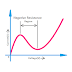## Definition and Concept

Negative resistance is a property of any device, component, or circuit that causes to decrease in current flow through them while voltage is increased across them. Basically, it creates exact opposite characteristics of VI characteristics of any conventional resistor. In a normal resistive element, when the voltage across it increases the current flow through it also increases but the negative resistance property makes it just the opposite.

Actually, the negative resistance comes from the differential or variable property. A conventional resistor does not change its resistance when all the physical quantities remain constant. But in the case of the negative resistance property, resistance increases with time at a certain condition.

Actually, the positive resistance and negative resistance both can be determined and measured by measuring voltage and current through an element or circuit. If the current flow increases with the increase of voltage then there may be a static resistance or positive resistance, but if the current flow decreases with the increase of voltage then there may be a negative resistance.

## Negative Resistance Examples

Here is the list of devices and components that can create negative resistance,
• Tunnel Diode
• Gunn Diode
• Thyristor
• Unijunction Transistor(UJT)
• IMPATT Diode
• Parametric Amplifiers
Negative resistance is not only created by the above devices. But it may happen due to some electrical or electromechanical, physical incidents such as,
• Electric ARC can create negative resistance
• Electric discharge through some types of gasses also can create differential negative resistance such as neon lamps, fluorescent lamps, etc.
• Negative resistance happens in some active electronic circuits such as amplifier circuits built with transistors, OpAmps, etc.

## Causes of Negative Resistance

Negative resistance is created with different principles in different devices.
• For example, In electric ARCs, gas discharge tubes, and SCRs, the negative resistance is created and controlled by the current flow, here voltage is the single-valued function of the current.
• On the other hand, in tunnel diodes, and Gunn diodes, negative resistance is controlled by the applied voltage not current.
• Semiconductor devices such as Field Effect Transistors use voltage to control the resistivity against the current flow through them but Bipolar Junction Transistors use current to control the resistivity.
• In some vacuum tubes, increasing of space charge with time clamps off the electrons flow, so a negative resistance occurs.
• The tunneling phenomenon causes to create negative resistance in the tunnel diode.

## Effects of Negative Resistance

In most of the cases, negative resistance is available in the form of differential or variable resistance. So it,
• Controls the current flow(generally it decreases the current flow)
• Help to consume less current and makes the system healthy.
• It helps to amplify the signal.
• Negative resistance can create microwave energy.

## Practical Applications of Negative Resistance

The negative resistance property is used to make amplifier circuits, oscillators, switching circuits, memory circuits, active filter circuits, etc.

Remember that there is no real negative resistor analogous to a conventional resistor that has a constant negative resistance over any range of voltage and current. Actually, the combination of internal physical property, applied voltage, and current conduction makes a system, device, or component to create negative resistance. And this manually created negative resistance is used for useful applications.

What is Negative Resistance? Examples, Causes, EffectsReviewed by Author on November 06, 2022 Rating: 5